Home | | Statistics 12th Std | Test of Significance for Two Normal Population Variances

# Test of Significance for Two Normal Population Variances

Test of Significance for Two Normal Population Variances: Procedure Steps, Example Solved Problems

TEST OF SIGNIFICANCE FOR TWO NORMAL POPULATION VARIANCES

Test procedure:

This test compares the variances of two independent normal populations, viz., N(μX, σX2)

and N(μY, σY2).

Step 1 : Null Hypothesis H0 : σX2 = σY2

That is, there is no significant difference between the variances of the two normal populations.

The alternative hypothesis can be chosen suitably from any one of the following

(i) H1 : σX2 < σY2 (ii) H1 : σX2 > σY2 (iii) H1 : σX2 ≠ σY2

Step 2 : Data

Let X1, X2,. . ., Xm and Y1, Y2,. . ., Yn be two independent samples drawn from two normal populations respectively.

Step 3 : Level of significance

α

Step 4 : The test Statistic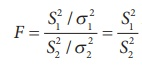under H0 and its sampling distribution under H0 is F(m-1, n-1).

Step 5 : Calculation of the Test Statistic

The test statisticStep 6 : Critical valuesStep 7 : Decision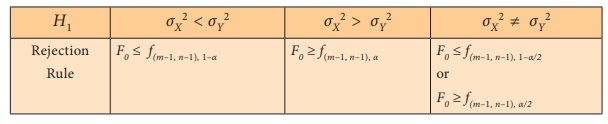Note 1: Since f(m–1, n–1),1-α  is not avilable in the given F-table, it is computed as the reciprocal of f(n–1, m–1).Note 2: A F-test is based on the ratio of variances, it is also known as Variance Ratio Test.

Note 3: When μX and μY are known, for testing the equality of variances of two normal populations, the test statistic isand follows Fm, n-distribution under H0

Example 3.1

Two samples of sizes 9 and 8 give the sum of squares of deviations from their respective means as 160 inches square and 91 inches square respectively. Test the hypothesis that the variances of the two populations from which the samples are drawn are equal at 10% level of significance.

Solution:

Step 1 : Null Hypothesis: H0 : σX2 = σY2

That is there is no significant difference between the two population variances.

Alternative Hypothesis: H1 : σX2 σY2

That is there is significant difference between the two population variances.

Step 2 : Data

m = 9, n = 8Step 3 : Level of significance

α = 10%

Step 4 : Test Statistic, under H0.

Step 5 : Calculation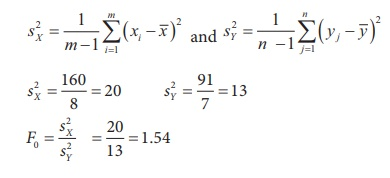Step 6 : Critical values

Since H1 is a two-sided alternative hypothesis the corresponding critical values are:Step 7 : Decision

Since f (8, 7),0.95 = 0.286 < F0 = 1.54 < f (8, 7),0.05 = 3.73, the null hypothesis is not rejected and we conclude that there is no significant difference between the two population variances.

Note 4: The critical values of F corresponding to α = 0.05 requires table values at 0.025 and 0.975 which are not provided. Hence α is taken as 0.1 in this example.

Example 3.2

A medical researcher claims that the variance of the heart rates (in beats per minute) of smokers is greater than the variance of heart rates of people who do not smoke. Samples from two groups are selected and the data is given below. Using = 0.05, test whether there is enough evidence to support the claim.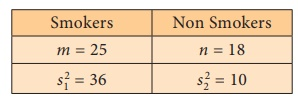Solution:

Step 1 : Null Hypothesis: H0 : σ12 = σ22

That is there is no significant difference between the two population variances.

H1 : σ12 > σ22

That is, the variance of heart rates of smokers is greater than that of non-smokers.

Step 2 : DataStep 3 : Level of significance α = 5%

Step 4 : Test statisticStep 5 : Calculation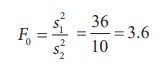Step 6 : Critical value

f (m-1,n-1),0.05 = f (24,17),0.05 = 2.19

Step 7 : Decision

Since F0 = 3.6 > f (24,17),0.05 = 2.19, the null hypothesis is rejected and we conclude that the variance of heart beats for smokers seems to be considerably higher compared to that of the non-smokers.

Example 3.3

The following table gives the random sample of marks scored by students in two schools, A and B.Is the variance of the marks of students in school A is less than that of those in school B?

Test at 5% level of significance.

Solution:

Let X1, X2 , …, Xm represent sample values for school A and let Y1, Y2, …, Yn represent sample values for school B.

Step 1 : Null Hypothesis: H1 : σX2 = σY2

That is, there is no significant difference between the two population variances.

Alternative Hypothesis: H1 : σX2 < σY2

That is, the variance of marks in school A is significantly less than that of school B.

Step 2 : Data

X1, X2,…, Xm are sample from school A

Y1, Y2, ..., Yn are sample from school B

Step 3 : Test statisticStep 4 : Calculations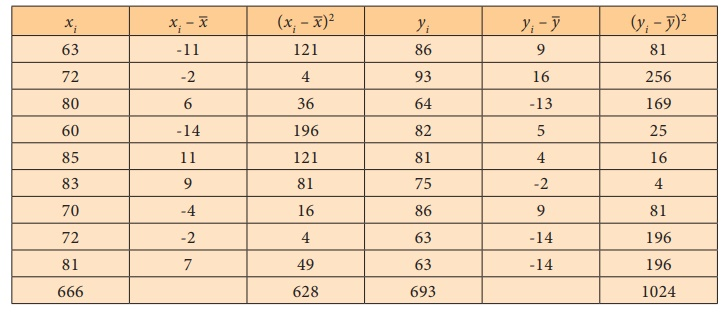Step 5 : Level of significance

= 5%

Step 6 : Critical value

f(9-1,9-1),0.95 = 1/ f( 8 ,8),0.05 = 1/3.44  = 0.291

Step 7 : Decision

Since F 0 = 0.609 > f(8,8),0.95 = 0.291, the null hypothesis is not rejected and we conclude that in school B there seems to be more variance present than in school A.

Tags : Procedure Steps, Example Solved Problems | Statistics , 12th Statistics : Chapter 3 : Tests Based on Sampling Distributions II
Study Material, Lecturing Notes, Assignment, Reference, Wiki description explanation, brief detail
12th Statistics : Chapter 3 : Tests Based on Sampling Distributions II : Test of Significance for Two Normal Population Variances | Procedure Steps, Example Solved Problems | Statistics